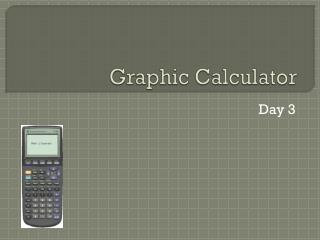Download PresentationGraphic CalculatorGraphic Calculator - PowerPoint PPT Presentation

Download PresentationGraphic Calculator
An Image/Link below is provided (as is) to download presentation

Download Policy: Content on the Website is provided to you AS IS for your information and personal use and may not be sold / licensed / shared on other websites without getting consent from its author. While downloading, if for some reason you are not able to download a presentation, the publisher may have deleted the file from their server.

- - - - - - - - - - - - - - - - - - - - - - - - - - - E N D - - - - - - - - - - - - - - - - - - - - - - - - - - -
Presentation Transcript

1. Graphic Calculator Day 3

2. Introduction Familiarize yourself with the graphing calculators and its features

3. End Products

4. Basic Skill: Correct Mode Press the ‘MODE’ button

5. Windows Dimension Press ‘WINDOW’ button Select Maximum and Minimum x- and y- values

6. Y= Key Press the ‘Y=’ button Write functions to create pictures Use your mathematical knowledge You can use up to 10 equations on the ‘Y=’ screen

7. Restricting domain Sometimes you may only require to graph a portion of the domain E.g. The function is y=x2 – 5 but it is only graphed for x≥1

8. Restricting domain Function Domain Type in the function in the parentheses, and divide the function by the restriction in the parentheses E.g. y=(x2 – 5)/{x≥1} The ≥ and ≤ symbols are found by hitting ‘2nd’ + ‘MATH’

9. GC Ex. 1 McDonald’s Sign

10. How many curves do you need? 4 Curves

11. y 4 x −2 0 Step 1 - Equations 0 What is the equation of this curve? y = - (x+2)2 + 8

12. Step 1 - Equations Find the equations & domains of ALL 4 curves and draw your McDonald’s sign To help you, here are the WINDOW setting:

13. GC Ex. 1 Solution

14. Step 2 - Turn off the axis ‘2nd’ + ‘ZOOM’ (‘FORMAT’)

15. Step 3 - Shading ‘2nd’ + ‘PRGM’ (‘DRAW’) 7: Shade(Y3 ,Y1) Y1: bottom Y equation, Y3: top Y equation Press ‘VARS’, move to ‘Y-VARS’ menu Press 1: Function Choose the equation

16. Step 4: Enter text ‘2nd’ + ‘PGRM’ (‘Draw’) 0: TEXT (ALPHA followed by letters)

17. Step 5: Storing picture ‘2nd’ + ‘PGRM’ (‘DRAW’) Use the arrow key to move to ‘STO’ menu 1: StorePic Key in a number from 1 to 9 Press Enter

18. Step 6: Recalling picture ‘2nd’ + ‘PRGM’ (‘DRAW’) Use the arrow key to move to ‘STO’ menu 2: RecallPic Key in the number where you store it Press Enter

19. Final product

20. GC Ex. 1 Macdonald’s Logo

21. GC Ex 2 Try it on your own…

22. Tips To restrict domain on both sides Use the ‘and’ function ‘2nd’ + ‘MATH’ (‘TEST’) Use your arrow key to move to ‘LOGIC’ menu 1: and E.g. -(X + 2)2 + 8/(X≥0) and (X≤10)

23. Tips To draw the Sun Equation of the circle: X2 + Y2 = r2

24. Drawing a straight vertical line Vertical lines cannot be graphed in function mode However, a very steep line can be produced What is y=x? Now, what is y=50x? …